# Load and boundary conditions

These records specify description of boundary conditions. The general format is following:

 *EntType (num#)(in) loadTimeFunction #(in) [valType #(in)] [dofs #(ia)] [isImposedTimeFunction #(in)]

The order of particular records is optional, boundary condition number is determined by (num#)(in) parameter. The numbering should start from one and should end at n, where n is the number of records. Time function value (given by loadTimeFunction parameter) is a multiplier, using which each component (value of loading or value of boundary condition) describes its time variation. The optional parameter valType allows to determine the physical meaning of bc value, which is sometimes required. Supported values are (1 - temperature, 2 - force/traction, 3 - pressure, 4 - humudity, 5 - velocity, 6 - displacement). Another optional parameter dofs is used to determine which dofs the boundary condition should act upon. It is not relevant for all BCs..

The nonzero value of isImposedTimeFunction time function indicates that given boundary condition is active, zero value indicates not active boundary condition in given time (the bc does not exist). By default, the boundary condition applies at any time.

Currently, EntType keyword can be one from

• Dirichlet boundary condition

 BoundaryCondition prescribedvalue #(rn) [d #(rn)]

Represents boundary condition. Prescribed value is specified using prescribedvalue parameter. The physical meaning of value is fully determined by corresponding DOF. Optionally, the prescribed value can be specified using d parameter. It is introduced for compatibility reasons. If prescribedvalue is specified, then d is ignored.

• Prescribed gradient boundary condition (Dirichlet type)

Prescribes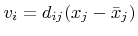or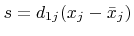where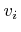are primary unknowns,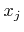is the coordinate of the node,is cCoords and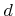is gradient. The parameter cCoords defaults to zero. This is typical boundary condition in multiscale analysis where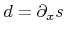would a macroscopic gradient at the integration point, i.e. this is a boundary condition for prolongation. It is also convenient to use when one wants to test a arbitrary specimen for shear.

• Mixed prescribed gradient / pressure boundary condition (Active type)

 MixedGradientPressure* devGradient #(ra) pressure #(rn) [cCoord #(ra)]

All boundary conditions of ensures that the deviatoric gradient and pressure is at least weakly fullfilled on the prescribed domain. They are used for computational homogenization of incompressible flow or elasticity problems.

• Mixed prescribed gradient / pressure boundary condition (Weakly periodic type)

Prescribes a periodic constant (unknown) stress tensor along the specified boundaries. For order set to 1, one obtains the same results as the Neumann boundary condition.

• Mixed prescribed gradient / pressure boundary condition (Neumann type)

Prescribes a constant (unknown) deviatoric stress tensor along the specified boundaries. Additional unknowns appears,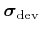, which is handled by the boundary condition itself (no control from the input file). The input devGradient is weakly fulfilled (homogenized over the elementsides). As with the the Dirichlet type, the volumetric gradient is free. This is useful in multiscale computations of RVE's that experience incompressible behavior, typically fluid problems. In that case, the element sides should cover the entire RVE boundary. It is also convenient to use when one wants to test a arbitrary specimen for shear, with a free volumetric part (in which case the pressure is set to zero). Symmetry is not assumed, so rigid body rotations are removed, but translations need to be prescribed separately.

• Mixed prescribed gradient / pressure boundary condition (Dirichlet type)

Prescribes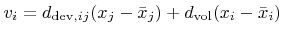, and a pressure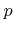. whereare primary unknowns,is the coordinate of the node,is cCoords and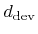is devGradient. The parameter cCoords defaults to zero. An additional unknown appears,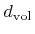, which is handled by the boundary condition itself (no control from the input file). This unknown is in a way related to the applied pressure. This is useful in multiscale computations of RVE's that experience incompressible behavior, typically fluid problems. It is also convenient to use when one wants to test a arbitrary specimen for shear, with a free volumetric part (in which case the pressure is set to zero).

• Nodal fluxes (loads)

 NodalLoad components #(ra) [cstype #(in)]

Concentrated nodal load. The components of nodal load vector are given by components parameter. The size of this vector corresponds to a total number of nodal DOFs, and i-th value corresponds to i-th DOF in associated dof manager. The load can be defined in global coordinate system (cstype = 0) or in entity - specific local coordinate system (cstype = 1, default).

•  PrescribedTractionPressureBC

Represents pressure boundary condition (of Dirichlet type) due to prescribed tractions. In CBS algorithm formulation the prescribed traction boundary condition leads indirectly to pressure boundary condition in corresponding nodes. This boundary condition implements this pressure bc. The value of bc is determined from applied tractions, that should be specified on element edges/surfaces using suitable boundary loads.

• Linear constraint boundary condition

 LinearConstraintBC weights #(ra) [weightsLtf #(ia)] dofmans #(in) dofs #(in) rhs #(rn) [rhsLtf #(in)] lhstype #(ia) rhsType #(ia)

This boundary condition implements a linear constraint in the form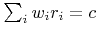, where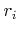are unknowns related to DOFs determined by dofmans and dofs, the weights are determined by weights and weightsLtf. The constant is determined by rhs and rhsLtf parameters. This boundary condition is introduced as additional stationary condition using Lagrange multiplier, which is an additional degree of freedom introduced by this boundary condition.

The individual DOFs are determined using dof manager numbers (dofmans array) and corresponding DOF indices (dofs). The weights corresponding to participating DOFs are specified using weights array. The weights are multiplied by value returned by load time function, associated to individual weight using optional weightsLtf array. By default, all weights are set to 1. The constant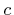is determined by rhs parameter and it is multiplied by the value of load time function, specified using rhsLtf parameter, or by 1 by default. The characteristic component, to which this boundary condition contributes must be identified using lhstype and rhsType parameters, values of which are corresponding to CharType enum. The left hand side contribution is assembled into terms identified by lhstype. The rhs contribution is assembled into the term identified by rhsType parameter. Note, that multiple values are allowed, this allows to select all variants of stifness matrix, for example. Note, that the size of dofmans, dofs, weights, weightsLtf arrays should be equal.

• InteractionBoundaryCondition

 InteractionBoundaryCondition

Is a special boundary condition prescribed on InteractionPFEMParticles 4.2 in the PFEM part of the FluidStructureProblem. This sort of particles is regarded as it would have prescribed velocities, but the values change dynamically, as the solid part deforms. The velocities are obtained from coupled structural nodes.

Subsections
Borek Patzak
2018-01-02